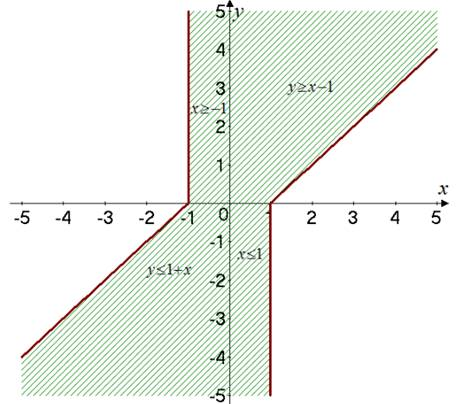# The sketch for the region in the plane that consists of all the points ( x , y ) such that | x − y | + | x | − | y | ≤ 2 .### Single Variable Calculus: Concepts...

4th Edition
James Stewart
Publisher: Cengage Learning
ISBN: 9781337687805### Single Variable Calculus: Concepts...

4th Edition
James Stewart
Publisher: Cengage Learning
ISBN: 9781337687805

#### Solutions

Chapter 1, Problem 10P
To determine

## To find: The sketch for the region in the plane that consists of all the points (x,y) such that |x−y|+|x|−|y|≤2 .

Expert Solution

### Explanation of Solution

Given:

The given condition is |xy|+|x||y|2 .

Calculation:

Consider the given condition is,

|xy|+|x||y|2

Consider the variable x as,

|x|={xifx0xifx<0

Consider the variable y as,

|y|={yify0yify<0

Then, consider the difference,

|xy|={xyifxy0xyifxy<0

Consider the case when x>0 and y<0 .

|xy|+|x||y|2xy+x(y)22x2x1

Consider the case when y>0 and x<0 .

|xy|+|x||y|2(xy)xy22x2x1

Consider the case when y>x , x>0 and y>0 .

|xy|+|x||y|2(xy)+xy202(true)

Consider the case when y<x , x>0 and y>0 .

|xy|+|x||y|2(xy)+xy2xy1y1

Consider the case when y<x , x<0 and y<0 .

|xy|+|x||y|2(xy)x(y)202(true)

Consider the case when y>x , x<0 and y<0 .

|xy|+|x||y|2(xy)x(y)22x+2y2y1+x

The graph for all the points in the region that consists of all the points x,y is shown in Figure 1Figure 1

### Have a homework question?

Subscribe to bartleby learn! Ask subject matter experts 30 homework questions each month. Plus, you’ll have access to millions of step-by-step textbook answers!